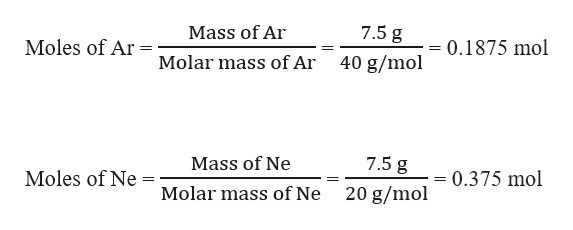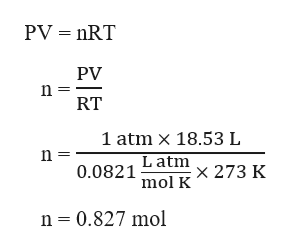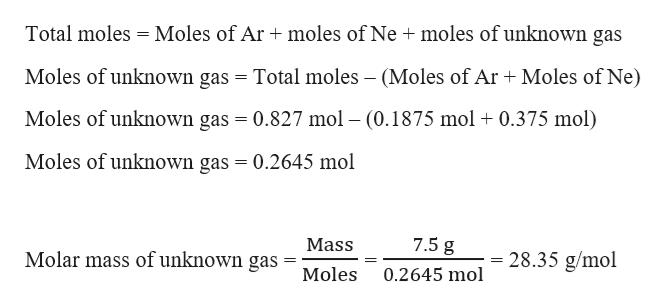# A gas mixture is made by combining 7.5 g each of Ar, Ne, and an unknown diatomic gas. At STP, the mixture occupies a volume of 18.53 L.What is the molar mass of the unknown gas?Identify the unknown gas.

Question
324 views

A gas mixture is made by combining 7.5 g each of Ar, Ne, and an unknown diatomic gas. At STP, the mixture occupies a volume of 18.53 L.

What is the molar mass of the unknown gas?

Identify the unknown gas.

check_circle

Step 1

Given,

Mass of Ar, Ne and an unknown diatomic gas = 7.5 g

Moles of Ar and Ne can be calculated as:help_outlineImage Transcriptionclose7.5 g Mass of Ar Moles of Ar -0.1875 mol Molar mass of Ar 40 g/mol 7.5 g Mass of Ne Moles of Ne = 0.375 mol Molar mass of Ne 20 g/mol fullscreen
Step 2

Volume, V = 18.53 L

At STP: Pressure, P = 1 atm and temperature, T = 273 K

The total number of moles in the gas mixture can be calculated using the ideal gas equation as:help_outlineImage TranscriptionclosePV nRT PV n RT 1 atm x 18.53 L Latm x 273 K 0.0821 mol K n 0.827 mol fullscreen
Step 3

The molar mass of the unknown g...help_outlineImage TranscriptioncloseTotal moles Moles of Ar moles of Ne moles of unknown gas Moles of unknown gas = Total moles - (Moles of Ar Moles of Ne) Moles of unknown gas 0.827 mol (0.1875 mol 0.375 mol) Moles of unknown gas = 0.2645 mol 7.5 g Mass 28.35 g/mol Molar mass of unknown gas - Moles 0.2645 mol fullscreen

### Want to see the full answer?

See Solution

#### Want to see this answer and more?

Solutions are written by subject experts who are available 24/7. Questions are typically answered within 1 hour.*

See Solution
*Response times may vary by subject and question.
Tagged in
ScienceChemistry

### Ideal gases